# Introduction

This file contains some examples of the `transfo` function to robustly transform variables toward central normality

``````library(cellWise)
library(robustHD) # for the TopGear data
``````

# Small toy example

We start with a small toy example, in which standard normal data with one NA is used.

``````set.seed(1)
X = rnorm(100)
X = NA
qqnorm(X)
``````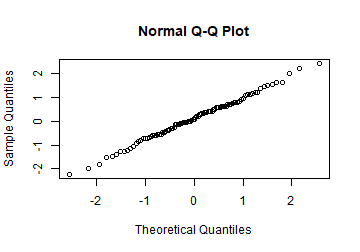We specify the transformation type as `"bestObj"`, which chooses between Box-Cox and Yeo-Johnson based on the value of the objective function. When there are negative values, Yeo-Johnson is chosen automatically. First, the classical MLE transformation is fit, indicated by the `robust = FALSE` argument:

``````ML.out <- transfo(X, type = "bestObj", robust=FALSE)
``````
``````##
##  The input data has 100 rows and 1 columns.
``````
``````ML.out\$lambdahat
``````
``````##  1.033255
``````

The value of the transformation parameter is close to 1, indicating no transformation is necesarry. Among the other outputs are the value of the objective function, the transformed data, estimates of the location and scale of the transformed data, and the standardized transformed data:

``````ML.out\$objective
``````
``````##  -139.9404
``````
``````ML.out\$Xt[45:55]
``````
``````##   -0.8681370 -0.8885374  0.2942895  0.7496632 -0.2364490         NA
##    0.3319026 -0.7845271  0.2679868 -1.3459716  1.5069813
``````
``````ML.out\$muhat
``````
``````##  0.0115562
``````
``````ML.out\$sigmahat
``````
``````##  0.9997822
``````
``````ML.out\$Zt[45:55]
``````
``````##   -0.8798848 -0.9002897  0.2827949  0.7382678 -0.2480593         NA
##    0.3204162 -0.7962567  0.2564864 -1.3578235  1.4957508
``````
``````qqnorm(ML.out\$Zt); abline(0,1)
``````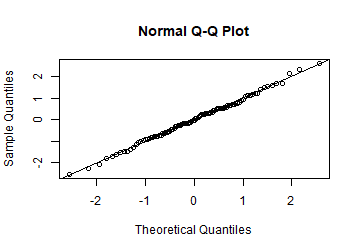Additionally, the function returns the weights of the observations and the transformation type that was used. The NA observation (number 50) gets weight zero:

``````plot(ML.out\$weights)
``````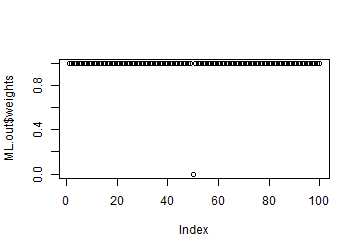``````ML.out\$ttypes
``````
``````##  "YJ"
``````

We can now repeat the same procedure with the robust estimator. This is specified using the `robust = TRUE` argument. The results do not differ too much from the classical MLE estimates on this dataset, since there are no outliers.

``````RewML.out <- transfo(X, type = "bestObj", robust=TRUE)
``````
``````##
##  The input data has 100 rows and 1 columns.
``````
``````RewML.out\$lambdahat
``````
``````##  1.099335
``````
``````RewML.out\$objective
``````
``````##  0.03190617
``````
``````RewML.out\$Xt[45:55]
``````
``````##   -0.8096768 -0.8290081  0.3225849  0.7847350 -0.2029893         NA
##    0.3604152 -0.7302972  0.2961708 -1.2590154  1.5696026
``````
``````RewML.out\$muhat
``````
``````##  0.03539293
``````
``````RewML.out\$sigmahat
``````
``````##  0.9554364
``````
``````RewML.out\$Zt[45:55]
``````
``````##   -0.8844857 -0.9047186  0.3005872  0.7842931 -0.2495009         NA
##    0.3401820 -0.8014036  0.2729412 -1.3547824  1.6057686
``````
``````qqnorm(RewML.out\$Zt); abline(0,1)
``````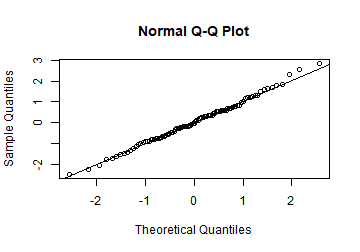Using the robust estimator, one large observation (number 61) also gets a weight of 0:

``````plot(RewML.out\$weights)
``````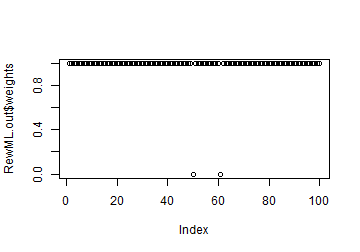``````X
``````
``````##  2.401618
``````
``````RewML.out\$ttypes
``````
``````##  "YJ"
``````

In order to illustrate the same concepts for the Box-Cox transformation, we take the exponential of the generated data. This yields a sample from a log-normal distribution.

``````X = exp(X)
``````

We first consider the classical maximum likelihood estimator. We obtain a transformation parameter close to 0, which corresponds with the logarithmic transformation.

``````ML.out <- transfo(X, type = "BC", robust=FALSE)
``````
``````##
##  The input data has 100 rows and 1 columns.
``````
``````ML.out\$lambdahat
``````
``````##  0.01965332
``````
``````ML.out\$objective
``````
``````##  8.401073
``````
``````ML.out\$Xt[45:55]
``````
``````##   -0.7576236 -0.7760807  0.2908451  0.6987220 -0.1865683         NA
##    0.3245717 -0.6819798  0.2672541 -1.1897966  1.3767554
``````
``````ML.out\$Zt[45:55]
``````
``````##   -0.8803983 -0.9009148  0.2850604  0.7384490 -0.2456236         NA
##    0.3225503 -0.7963140  0.2588371 -1.3607939  1.4921386
``````
``````qqnorm(ML.out\$Zt); abline(0,1)
``````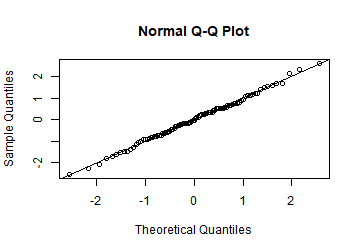The weights are again all equal to 1, except for the NA (number 50) which gets weight zero:

``````plot(ML.out\$weights)
````````````ML.out\$ttypes
``````
``````##  "BC"
``````

We now repeat the example with the robust transformation. The estimated transformation parameter is again fairly close to 0, as expected.

``````RewML.out <- transfo(X, type = "bestObj", robust=TRUE)
``````
``````##
##  The input data has 100 rows and 1 columns.
``````
``````RewML.out\$lambdahat
``````
``````##  0.1143505
``````
``````RewML.out\$objective
``````
``````##  0.03654314
``````
``````RewML.out\$Xt[45:55]
``````
``````##   -0.7309555 -0.7481103  0.2948796  0.7222461 -0.1849279         NA
##    0.3296004 -0.6603303  0.2706587 -1.1247315  1.4696529
``````
``````RewML.out\$Zt[45:55]
``````
``````##   -0.8926229 -0.9129574  0.3233614  0.8299447 -0.2453834         NA
##    0.3645181 -0.8089065  0.2946509 -1.3593892  1.7158910
``````
``````qqnorm(RewML.out\$Zt); abline(0,1)
``````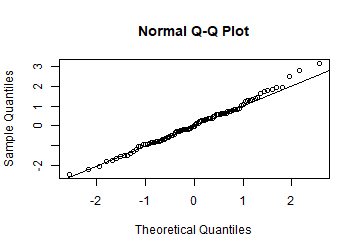``````RewML.out\$ttypes
``````
``````##  "BC"
``````

# TopGear example

This dataset is part of the `robustHD` package. We use two of its variables to illustrate the effect outliers can have on estimating a transformation using maximum likelihood. The robust estimators yield more reasonable results.

``````data(TopGear)
``````

First, we do some preprocessing to avoid zeroes.

``````CD.out   <- checkDataSet(TopGear)
``````
``````##
##  The input data has 297 rows and 32 columns.
##
##  The input data contained 19 non-numeric columns (variables).
##  Their column names are:
##
##   Maker              Model              Type               Fuel
##   Automatic          Bluetooth          ClimateControl     CruiseControl
##  ElectricSeats      Leather            ParkingSensors     PowerSteering
##  SatNav             ESP                Origin
##
##  These columns will be ignored in the analysis.
##  We continue with the remaining 13 numeric columns:
##
##   Price        Cylinders    Displacement BHP          Torque
##   Acceleration TopSpeed     MPG          Weight       Length
##  Width        Height       Verdict
##
##  The data contained 1 rows with over 50% of NAs.
##  Their row names are:
##
##  70
##
##  These rows will be ignored in the analysis.
##  We continue with the remaining 296 rows:
##
##    1   2   3   4   5   6   7   8   9   10  11  12  13  14  15  16  17  18
##   19  20  21  22  23  24  25  26  27  28  29  30  31  32  33  34  35  36
##   37  38  39  40  41  42  43  44  45  46  47  48  49  50  51  52  53  54
##   55  56  57  58  59  60  61  62  63  64  65  66  67  68  69  71  72  73
##   74  75  76  77  78  79  80  81  82  83  84  85  86  87  88  89  90  91
##   92  93  94  95  96  97  98  99  100 101 102 103 104 105 106 107 108 109
##  110 111 112 113 114 115 116 117 118 119 120 121 122 123 124 125 126 127
##  128 129 130 131 132 133 134 135 136 137 138 139 140 141 142 143 144 145
##  146 147 148 149 150 151 152 153 154 155 156 157 158 159 160 161 162 163
##  164 165 166 167 168 169 170 171 172 173 174 175 176 177 178 179 180 181
##  182 183 184 185 186 187 188 189 190 191 192 193 194 195 196 197 198 199
##  200 201 202 203 204 205 206 207 208 209 210 211 212 213 214 215 216 217
##  218 219 220 221 222 223 224 225 226 227 228 229 230 231 232 233 234 235
##  236 237 238 239 240 241 242 243 244 245 246 247 248 249 250 251 252 253
##  254 255 256 257 258 259 260 261 262 263 264 265 266 267 268 269 270 271
##  272 273 274 275 276 277 278 279 280 281 282 283 284 285 286 287 288 289
##  290 291 292 293 294 295 296 297
##
##  The data contained 1 columns with zero or tiny median absolute deviation.
##  Their column names are:
##
##  Cylinders
##
##  These columns will be ignored in the analysis.
##  We continue with the remaining 12 columns:
##
##   Price        Displacement BHP          Torque       Acceleration
##   TopSpeed     MPG          Weight       Length       Width
##  Height       Verdict
##
##  The final data set we will analyze has 296 rows and 12 columns.
##
``````
``````colnames(CD.out\$remX)
``````
``````##   "Price"        "Displacement" "BHP"          "Torque"       "Acceleration"
##   "TopSpeed"     "MPG"          "Weight"       "Length"       "Width"
##  "Height"       "Verdict"
``````
``````# remove the subjective variable `Verdict':
X        <- CD.out\$remX[,-12]
carnames <- TopGear[CD.out\$rowInAnalysis, 1:2]
X        <- pmax(X, 1e-8) # avoids zeroes
``````

Now we estimate transformation parameters (takes under a second) using classical MLE as well as the reweighted MLE which is much more robust.

``````lambdarange <- c(-4, 6) # for illustration, is the default anyway
ML.out    <- transfo(X, lambdarange = lambdarange, type = "BC", robust=F)
``````
``````##
##  The input data has 296 rows and 11 columns.
``````
``````RewML.out <- transfo(X, lambdarange = lambdarange, type = "BC", robust=T)
``````
``````##
##  The input data has 296 rows and 11 columns.
``````

Now consider the transformation of the variable `MPG`.

``````ML.out\$lambdahat
``````
``````##  -0.1077643
``````
``````RewML.out\$lambdahat
``````
``````##  0.8360462
``````

The classical method estimates a transformation parameter of -0.11, which is close to the log transform. The robust method barely transforms the original variable with a transformation parameter of 0.84, relatively close to 1. The QQplots below suggest that the robust transformation is much more reasonable:

``````qqnorm(X[, 7], main = "", cex.lab = 1.5, cex.axis = 1.5)
``````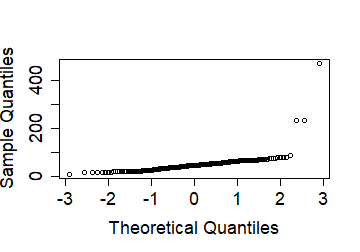``````qqnorm(ML.out\$Zt[, 7], main = "Classical transform",
cex.lab = 1.5, cex.axis = 1.5)
abline(0, 1)
``````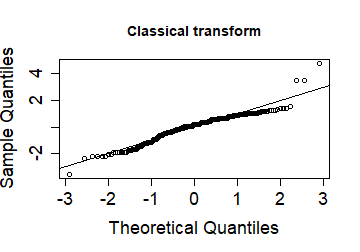``````qqnorm(RewML.out\$Zt[, 7], main = "Robustly transformed",
cex.lab = 1.5, cex.axis = 1.5)
abline(0, 1)
``````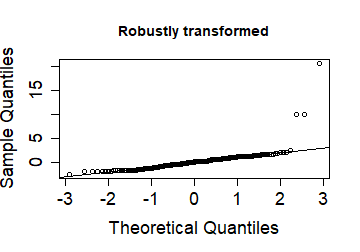Now consider the transformation of the variable `Weight`.

``````ML.out\$lambdahat
``````
``````##  0.8260084
``````
``````RewML.out\$lambdahat
``````
``````##  0.09032881
``````

Here, the situation is reversed: the classical estimator finds a transformation parameter (0.83) relatively close to one, whereas the robust transformation finds a parameter (0.09) close to the log transform. The QQplots below again suggest that the robust transformation is much more reasonable:

``````qqnorm(X[, 8], main = "Original variable",
cex.lab = 1.5, cex.axis = 1.5)
``````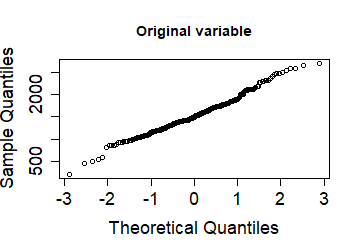``````qqnorm(ML.out\$Zt[, 8], main = "Classical transform",
cex.lab = 1.5, cex.axis = 1.5)
abline(0, 1)
``````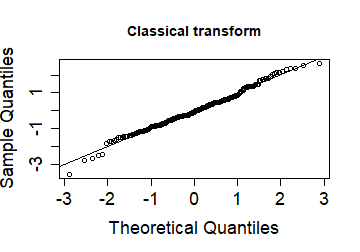``````qqnorm(RewML.out\$Zt[, 8], main = "Robustly transformed",
cex.lab = 1.5, cex.axis = 1.5)
abline(0, 1)
``````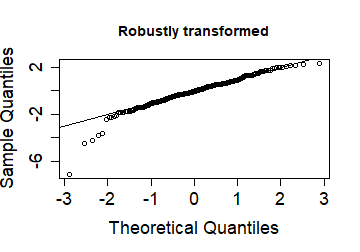The classical transformation does not fit as well as it attempts to push the 5 outliers into the fold at the expense of creating skewness in the center of the data.

# Glass data example

In this example we study the glass data. It consists of spectra with 750 wavelengths of 180 archaeological glass samples.

``````data("glass")
``````

First, we do some preprocessing to avoid variables with very small scales (in fact, the first 13 variables have mad equal to zero). Afterwards, we focus on the first 500 wavelengths since this is where most of the activity occurs.

``````X <- as.matrix(glass[, -c(1:13)])
X <- X[, 1:500]
Z <- scale(X, center=FALSE, robustbase::colMedians(X))
dim(Z)
``````
``````##  180 500
``````

Now we estimate transformation parameters using classical MLE as well as the reweighted MLE which is much more robust. This only takes a few seconds for the 500 variables.

``````ML.out    <- transfo(Z, type = "YJ", robust=F)
``````
``````##
##  The input data has 180 rows and 500 columns.
``````
``````RewML.out <- transfo(Z, type = "YJ", robust=T)
``````
``````##
##  The input data has 180 rows and 500 columns.
``````

We now construct the cellmaps:

``````indcells_clas = which(abs(ML.out\$Zt) > sqrt(qchisq(0.99, 1)))
indcells_rob  = which(abs(RewML.out\$Zt) > sqrt(qchisq(0.99, 1)))
n = dim(ML.out\$Zt)
d = dim(ML.out\$Zt); d
``````
``````##  500
``````
``````nrowsinblock = 5
rowlabels = rep("", floor(n/nrowsinblock));
rowtitle = ""
ncolumnsinblock = 5
columnlabels = rep("",floor(d/ncolumnsinblock));
columnlabels = "1";
columnlabels = "wavelengths";
columnlabels[floor(d/ncolumnsinblock)] = "500"
columntitle = ""

CM_clas = cellMap(D = ML.out\$Zt, R = ML.out\$Zt,
indcells = indcells_clas,
indrows = NULL,
rowlabels = rowlabels,
columnlabels = columnlabels,
mTitle = "YJ transformed variables by ML",
rowtitle = rowtitle,
columntitle = columntitle,
nrowsinblock = nrowsinblock,
ncolumnsinblock = ncolumnsinblock,
columnangle = 0,
autolabel = FALSE,
colContrast = 1.0,
drawCircles = F)
plot(CM_clas)
``````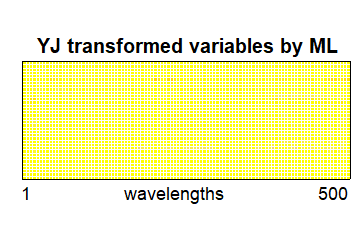``````CM_rob = cellMap(D = RewML.out\$Zt, R = RewML.out\$Zt,
indcells = indcells_rob,
indrows = NULL,
rowlabels = rowlabels,
mTitle = "YJ transformed variables by RewML",
columnlabels = columnlabels,
rowtitle = rowtitle,
columntitle = columntitle,
nrowsinblock = nrowsinblock,
ncolumnsinblock = ncolumnsinblock,
columnangle = 0,
autolabel = FALSE,
colContrast = 1.0,
drawCircles = F)
plot(CM_rob)
``````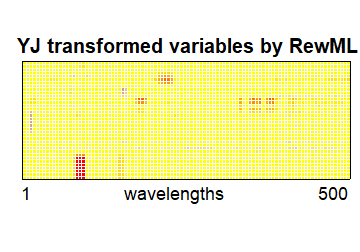``````# pdf("Glass_YJ_ML_RewML.pdf",width=10,height=6)
# gridExtra::grid.arrange(CM_clas, CM_rob,ncol=1)
# dev.off()
``````

# DPOSS data example

As a last example, we analyze the DPOSS data. This is a random subset of 20'000 stars from the Digitized Palomar Sky Survey described by Odewahn et al (1998).

``````data("dposs") # in package cellWise
n = nrow(dposs); n
``````
``````##  20000
``````
``````ncol(dposs)
``````
``````##  21
``````

There are lots of missing values in this dataset. Therefore, we first do some preprocessing to select the band of wavelengths which contain the fewest missing values.

``````missmat = is.na(dposs)
sizemat = nrow(missmat)*ncol(missmat); sizemat
``````
``````##  420000
``````
``````100*sum(as.vector(missmat))/sizemat
``````
``````##  50.20952
``````
``````missrow = length(which(rowSums(missmat) > 0))
100*missrow/nrow(missmat)
``````
``````##  84.61
``````
``````# Missingness by band:

# F band:
300*sum(as.vector(missmat[,1:7]))/sizemat
``````
``````##  44.75
``````
``````100*length(which(rowSums(missmat[,1:7]) > 0))/20000
``````
``````##  44.75
``````
``````# J band:
300*sum(as.vector(missmat[,8:14]))/sizemat
``````
``````##  42.43857
``````
``````100*length(which(rowSums(missmat[,8:14]) > 0))/20000
``````
``````##  42.61
``````
``````# N band:
300*sum(as.vector(missmat[,15:21]))/sizemat
``````
``````##  63.44
``````
``````100*length(which(rowSums(missmat[,15:21]) > 0))/20000
``````
``````##  63.44
``````
``````# So typically the whole band is missing or not.
# We focus on the J band which has the most available rows.

indx = which(rowSums(missmat[,8:14]) ==0)
dpossJ = dposs[indx,8:14]
dim(dpossJ)
``````
``````##  11478     7
``````

A quick exploration of the skewness in the data shows that there is skewness in both directions:

``````par(mfrow = c(1, 1))
boxplot(scale(dpossJ))
``````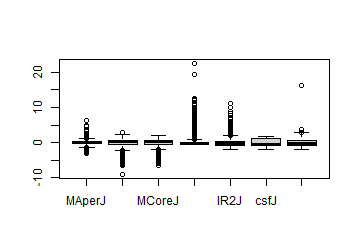Now we fit the Yeo-Johnson transformation robustly and transform the data with the estimated parameters. The analysis shows that there are both lambdas larger than 1 and smaller than 1, corresponding with the skewness in both directions.

``````transfoJ_YJ <- transfo(dpossJ, type = "YJ", robust = T)
``````
``````##
##  The input data has 11478 rows and 7 columns.
``````
``````plot(transfoJ_YJ\$lambdahat)
``````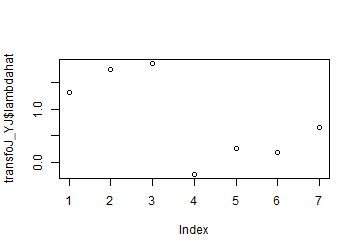``````dpossJ_YJ = transfoJ_YJ\$Xt
``````

We now apply cellwise robust PCA to further analyze the data. We perform the PCA using both the original and the transformed data.

``````DDCPars = list(fastDDC=F,fracNA=0.5)
MacroPCAPars = list(DDCpars=DDCPars,scale=TRUE,silent=T)
``````

Let's make a plot of the scores of YJ-transformed data:

``````MacroPCAdpossJ_YJ\$scores[, 1] <- -MacroPCAdpossJ_YJ\$scores[, 1]
cols <- rep("black", dim(MacroPCAdpossJ\$scores))
cols[c(98, 10894)] <- "darkorange"
cols[which(MacroPCAdpossJ_YJ\$SD > 14)] <- "skyblue3"
cols[which(MacroPCAdpossJ_YJ\$SD > 25)] <- "firebrick"

# pdf("dpossJ_scores_YJ.pdf")
``````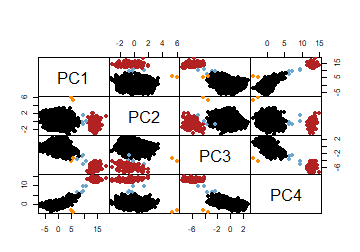``````# dev.off()
``````

We finally show the outlier maps on the transformed and the untransformed data:

``````# pdf("dpossJ_outliermap_YJ.pdf",height=5,width=5)
outlierMap(MacroPCAdpossJ_YJ, title="Outlier map of transformed data", col=cols, labelOut=FALSE)
``````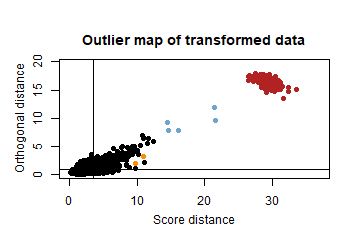``````# dev.off()

# pdf("dpossJ_outliermap_rawdata.pdf",height=5,width=5)
outlierMap(MacroPCAdpossJ, title="Outlier map of raw data", col=cols, labelOut=FALSE)
``````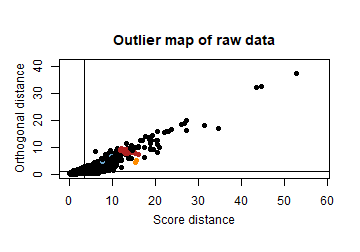``````# dev.off()
``````